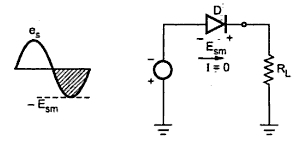### Half Wave Rectifier : Part3

1.11 Peak Inverse voltage (PIV)
The peak Inverse Voltage is the peak voltage across the diode in the reverse direction i.e. when the diode is reverse biased. In half wave rectifier, the load current is ideally zero when the diode is reverse biased and hence the maximum value of the voltage that can exist across the diode is nothing but Esm. This is shown in the Fig. 1.Fig. 1  PIV rating of diode

Thus PIV occurs at the peak of each negative half cycle of the input, when diode is reverse biased and not conducting.
This is called PIV rating of a diode. So diode must be selected based on this PIV rating and the circuit specifications.
1.12  Transformer Utilization Factor (T.U.F)
The factor which indicates how much is the utilization of the transformer in the circuit is called Transformer Utilization Factor (T.U.F.)
Definition :
The T.U.F. is defined as the ratio of d.c. power delivered to the load to the a.c. power rating of the transformer. While calculating the a.c. power rating, it is necessary to consider r.m.s. value a.c. voltage and current.
The T.U.F. for half wave rectifier can be obtained as,
Remember that the secondary voltage is purely sinusoidal hence its r.m.s. value is 1/√2 times maximum while the current is half sinusoidal hence its r.m.s. value is 1/2 of the maximum, as derived earlier.
Neglecting the drop across Rf and Rs we can write,
Note : The value of T.U.F. is low which shows that in half wave circuit, the transformer is not filly utilized.
1.3 Voltage Regulation
The secondary voltage should not change with respect to the load current. The voltage regulation is the factor which tells us about the change in the d.c. output voltage as load changes from no load to full load condition.
If  (Vdc)NL = D.C. voltage on no load
(Vdc)FL  = D.C. voltage on full load
then voltage regulation is defined as,
Note : Less the value of voltage regulation, better is the performance of rectifier circuit. For a half wave circuit,
Neglecting winding resistance,
Note : Ideally the load regulation is zero as ideal value of Rf is zero in forward biased condition.
1.13.1 Regulation Characteristics
Consider the equivalent circuit of the half wave rectifier, for positive half cycle of the transformer secondary voltage as shown in the Fig. 2(a).
As load increases (RL decreases), the drop across Rs and Rf goes on increasing, but the transformer, secondary voltage remains same. Hence the d.c. output voltage decreases. Hence load voltage decreases as load changes from no load to full load. To keep the drop across Rs and  Rf minimum, values of Rs and Rf must be as small as possible. The graph of load voltage against load current is called regulation characteristics which is drooping in nature in the Fig. 2(b).Fig. 2

1.14 Disadvantages of Half Wave Rectifier Circuit
The various disadvantages of the half wave rectifier circuit are,
1. The ripple factor of half wave rectifier circuit is 1.21, which is quite high. The output contains lot of varying components.
2. The maximum theoretical rectification efficiency is found to be 40 %. The practical value will be less than this. This indicates that half wave rectifier circuit is quite inefficient.
3. The circuit has low transformer utilization factor, showing that the transformer is not fully utilized.
4. The d.c. current is flowing through the secondary winding of the transformer which may cause d.c. saturation of the core of the transformer. To minimize the saturation, transformer size have to be increased accordingly. This increases the cost.
Because of all this disadvantages, the half-wave rectifier circuit is normally not used as a power rectifier circuit.

Examples on half wave rectifier